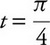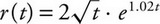# AP Calculus AB Practice Test 50

### Test Information7 questions21 minutes

Calculator Allowed

1. A wire 40 centimeters long is being cut into two pieces to make a circle and a square. If the total area of the circle and the square is a minimum, how much wire, in centimeters, should be used for the circle?

2. Given that f(x) = arccos(2x), what is the slope of the tangent to the graph of fat x= 0?

3. A solid is generated by revolving, about the x-axis, the region bounded by the curves y= x3- 2x+ 1 and y= x+ 2 in the second quadrant. The volume of the solid is

4. A function fis given as f(x) = x3+ 1 for 1 ≤ x≤ 3. If 1 ≤ c≤ 3 and f′(c) is equal to the average rate of change of fon the interval [1, 3], what is the value of c?

5. The velocity of a particle moving on a straight line is given as v(t) = cos(t)(esin(t)) for t≥ 0. At, what is the acceleration of the particle?

6. The region, in the first quadrant, bounded by the graph of y= cos(x), the x-axis, and the y-axis, is divided by the line x= kinto two regions of equal area. What is the value of k?

7. In a given petri dish, the number of bacteria grows at approximately the rate ofbacteria per day, wheretis the number of days since the culture in the petri dish began. At day two, there are 150 bacteria. Which of the following is the best approximation for the number of bacteria at day five?